## Tamilnadu Samacheer Kalvi 6th Maths Solutions Term 3 Chapter 2 Integers Intext Questions

(Try These Text Book Page No. 26)

Question 1.
(i) + 24
(ii) -13
(iii) -9
(iv) 8
Solution:
(i) + 24 is read as ‘ plus 24’ or ‘positive 24’
(ii) -13 is read as ‘minus 13’ or ‘negative 13’
(iii) -9 is read as ‘minus 9’ or ‘ negative 9’
(iv) 8 is read as ‘plus 8’ or ‘positive 8’ or simply ‘8’

Question 2.
Draw a number line and mark the following integers.
(i) 0
(ii) -6
(iii) 5
(iv) -8
Solution: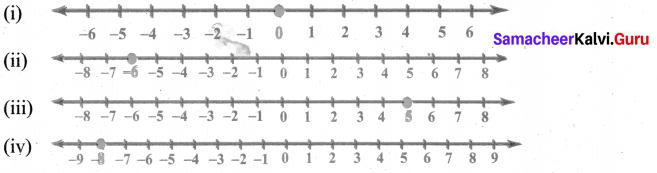Question 3.
Are all natural numbers integers?
Solution:
Yes, all natural numbers are positive integersQuestion 4.
Which part of the integers are not whole numbers?
Solution:
The numbers 0, 1, 2, 3, are whole numbers. Therefore negative part of the integers are not whole numbers.

Question 5.
How many units should you move to the left of 3 to reach -4.
Solution: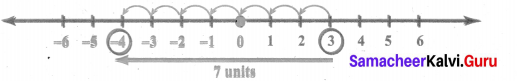From 3, we should move 7 units to the left to reach -4.

(Activity Textbook Page No. 27)

Question 1.
Ask your parents / grandparents about the depth at which the various types of vegetables (seeds) should be planted, for their better and efficient growth. For the same, draw a number line indicating the depth of various vegetable seeds. (Draw the planting chart!).
Solution:
Activity to be done by the students themselves

(Try These Text Book Page No. 29)

Question 1.
Find the opposite of the following numbers :
(i) 55
(ii) -300
(iii) +5080
(iv) -2500
(v) 0
Solution:
For every positive integer, there is a corresponding negative integer as its opposite number and vice versa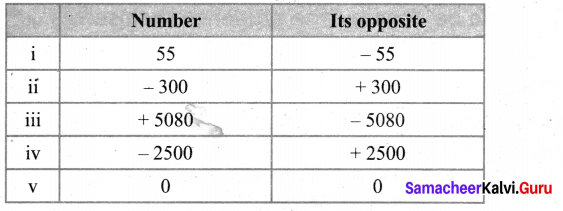Question 2.
Represent the following situations as integers.
i) A loss of ₹ 2000
iii) Fishes found at 60 m below the sea level
iv) 18°C below 0°C
v) Gaining 13 points
vi) A jet plane at a height of 2500 m
Solution:
(i) Since loss is negative, a loss of t 2000 is denoted as – ₹ 2000
(ii) Here CE (AD) can be considered as a positive number. Hence CE (AD) 2018 can be represented as + 2018.
(iii) Below sea level is represented as -60 m.
(iv) 18°C below 0°C is represented as – 18°C
(v) Gaining 13 points can be represented as + 13
(vi) Height of 2500 m is represented as + 2500 m.Question 3.
Suppose in a building, there are 2 basement floors. If the ground floor is denoted as zero, how can we represent the basement floors?
Solution:
If the ground floor is denoted as 0, basement floors are represented as -1 and -2.

(Try This Textbook Page No. 31)

Question 1.
For two numbers, say 3 and 5, we know that 5 > 3. Will there be a change in the inequality if both the numbers have negative sign before them?
Solution:
We know that 5 > 3
But when we change the sign of both the numbers we get – 5 and – 3.
Here -5 is 2 units to the left of-3 and so – 5 < 3.
∴ The inequality changes when the signs of both the numbers are changed.

(Activity Textbook Page No. 31)

Question 1.
Take two cards from a deck of playing cards and identify, which is greater between them, assuming that the Joker card represents zero, black cards represent positive integers, red cards represent negative integers and the cards A, J, Q and K represent 1,11,12 and 13 respectively.
Solution:
Activity to be done by the students themselves

(Try These Textbook Page No. 31)

Question 1.
Is -15 < -26? Why?
Solution:
Given statement is wrong.
We will draw the number line and plot the numbers on it.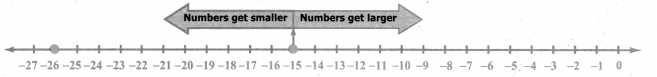So -26 < -15

Question 2.
Which is smaller -3 or -5? Why?
Solution:
Comparing the numbers from the number line.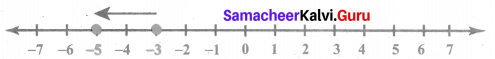– 5 is 2 units to the left of -3 and so -5 is smaller than -3. i.e. – 5 < – 3

Question 3.
Which is greater 7 or -4? Why?
Solution:
7 is a positive integer and lies to the right of 0.-4 is a negative integer and lies to the left of 0
∴ 7 > -4

Question 4.
Which is the greatest negative integer?
Solution: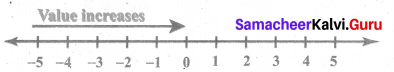– 1 is the greatest negative integer.Question 5.
Which is the smallest positive integer?
Solution: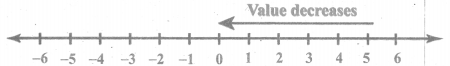+ 1 is the smallest positive integer.